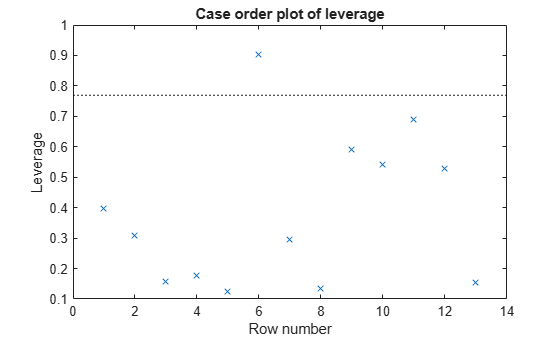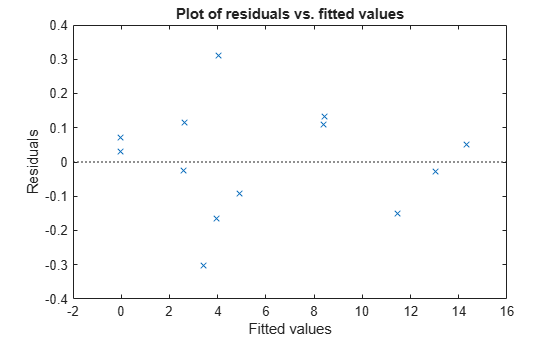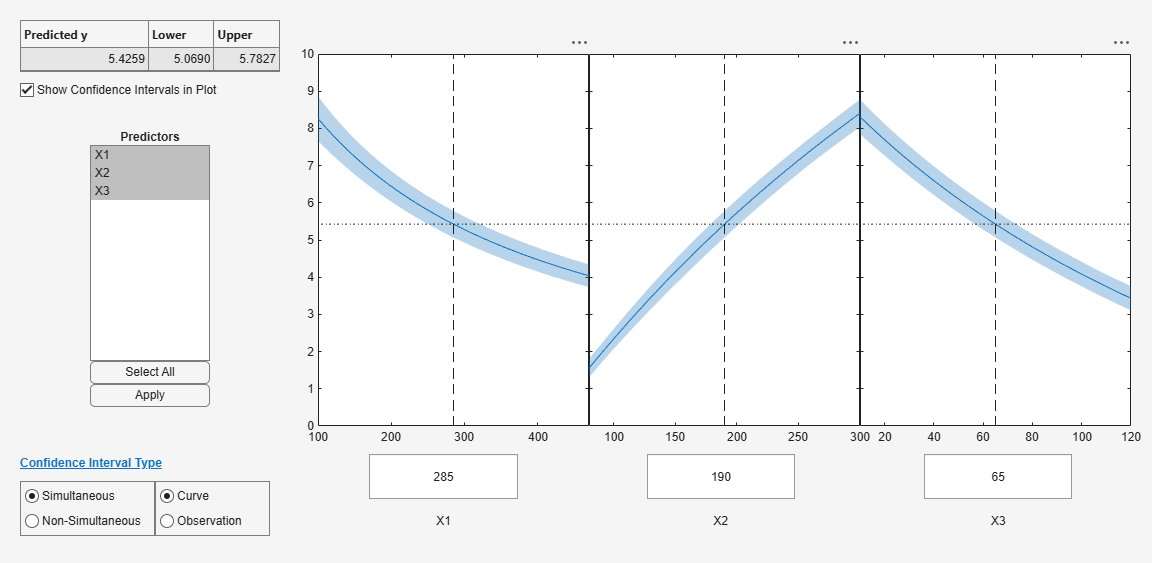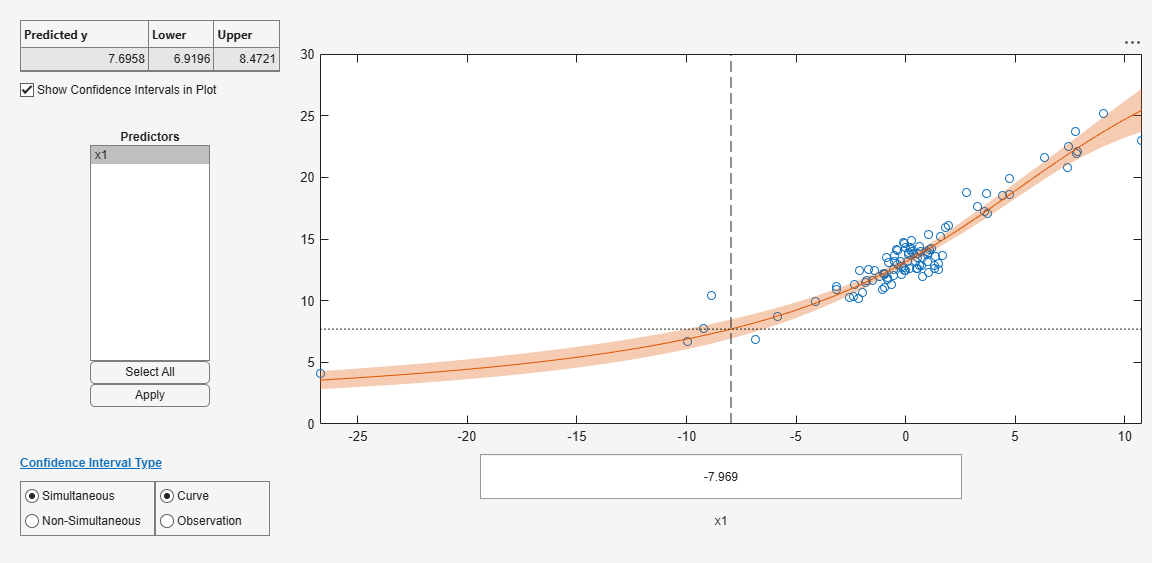Main Content

## Nonlinear Regression

### What Are Parametric Nonlinear Regression Models?

Parametric nonlinear models represent the relationship between a continuous response variable and one or more continuous predictor variables in the form

y = f(X,β) + ε,

where

• y is an n-by-1 vector of observations of the response variable.

• f is any function of X and β that evaluates each row of X along with the vector β to compute the prediction for the corresponding row of y.

• X is an n-by-p matrix of predictors, with one row for each observation, and one column for each predictor.

• β is a p-by-1 vector of unknown parameters to be estimated.

• ε is an n-by-1 vector of independent, identically distributed random disturbances.

In contrast, nonparametric models do not attempt to characterize the relationship between predictors and response with model parameters. Descriptions are often graphical, as in the case of Decision Trees.

`fitnlm` attempts to find values of the parameters β that minimize the mean squared differences between the observed responses y and the predictions of the model f(X,β). To do so, it needs a starting value `beta0` before iteratively modifying the vector β to a vector with minimal mean squared error.

### Prepare Data

To begin fitting a regression, put your data into a form that fitting functions expect. All regression techniques begin with input data in an array `X` and response data in a separate vector `y`, or input data in a table or dataset array `tbl` and response data as a column in `tbl`. Each row of the input data represents one observation. Each column represents one predictor (variable).

For a table or dataset array `tbl`, indicate the response variable with the `'ResponseVar'` name-value pair:

`mdl = fitlm(tbl,'ResponseVar','BloodPressure');`

The response variable is the last column by default.

You cannot use categorical predictors for nonlinear regression. A categorical predictor is one that takes values from a fixed set of possibilities.

Represent missing data as `NaN` for both input data and response data.

#### Dataset Array for Input and Response Data

For example, to create a dataset array from an Excel® spreadsheet:

```ds = dataset('XLSFile','hospital.xls',... 'ReadObsNames',true);```

To create a dataset array from workspace variables:

```load carsmall ds = dataset(Weight,Model_Year,MPG);```

#### Table for Input and Response Data

To create a table from an Excel spreadsheet:

```tbl = readtable('hospital.xls',... 'ReadRowNames',true);```

To create a table from workspace variables:

```load carsmall tbl = table(Weight,Model_Year,MPG);```

#### Numeric Matrix for Input Data and Numeric Vector for Response

For example, to create numeric arrays from workspace variables:

```load carsmall X = [Weight Horsepower Cylinders Model_Year]; y = MPG;```

To create numeric arrays from an Excel spreadsheet:

```[X, Xnames] = xlsread('hospital.xls'); y = X(:,4); % response y is systolic pressure X(:,4) = []; % remove y from the X matrix```

Notice that the nonnumeric entries, such as `sex`, do not appear in `X`.

### Represent the Nonlinear Model

There are several ways to represent a nonlinear model. Use whichever is most convenient.

The nonlinear model is a required input to `fitnlm`, in the `modelfun` input.

`fitnlm` assumes that the response function f(X,β) is smooth in the parameters β. If your function is not smooth, `fitnlm` can fail to provide optimal parameter estimates.

#### Function Handle to Anonymous Function or Function File

The function handle @`modelfun``(b,x)` accepts a vector `b` and matrix, table, or dataset array `x`. The function handle should return a vector `f` with the same number of rows as `x`. For example, the function file `hougen.m` computes

`$\text{hougen}\left(b,x\right)=\frac{b\left(1\right)x\left(2\right)-x\left(3\right)/b\left(5\right)}{1+b\left(2\right)x\left(1\right)+b\left(3\right)x\left(2\right)+b\left(4\right)x\left(3\right)}.$`

Examine the function by entering `type hougen` at the MATLAB® command line.

```function yhat = hougen(beta,x) %HOUGEN Hougen-Watson model for reaction kinetics. % YHAT = HOUGEN(BETA,X) gives the predicted values of the % reaction rate, YHAT, as a function of the vector of % parameters, BETA, and the matrix of data, X. % BETA must have 5 elements and X must have three % columns. % % The model form is: % y = (b1*x2 - x3/b5)./(1+b2*x1+b3*x2+b4*x3) % % Reference: %  Bates, Douglas, and Watts, Donald, "Nonlinear % Regression Analysis and Its Applications", Wiley % 1988 p. 271-272. % Copyright 1993-2004 The MathWorks, Inc. % B.A. Jones 1-06-95. b1 = beta(1); b2 = beta(2); b3 = beta(3); b4 = beta(4); b5 = beta(5); x1 = x(:,1); x2 = x(:,2); x3 = x(:,3); yhat = (b1*x2 - x3/b5)./(1+b2*x1+b3*x2+b4*x3);```

You can write an anonymous function that performs the same calculation as `hougen.m`.

```modelfun = @(b,x)(b(1)*x(:,2) - x(:,3)/b(5))./... (1 + b(2)*x(:,1) + b(3)*x(:,2) + b(4)*x(:,3));```

#### Text Representation of Formula

For data in a matrix `X` and response in a vector `y`:

• Represent the formula using `'x1'` as the first predictor (column) in `X`, `'x2'` as the second predictor, etc.

• Represent the vector of parameters to optimize as `'b1'`, `'b2'`, etc.

• Write the formula as `'y ~ (mathematical expressions)'`.

For example, to represent the response to the reaction data:

`modelfun = 'y ~ (b1*x2 - x3/b5)/(1 + b2*x1 + b3*x2 + b4*x3)';`

For data in a table or dataset array, you can use formulas represented as the variable names from the table or dataset array. Put the response variable name at the left of the formula, followed by a `~`, followed by a character vector representing the response formula.

This example shows how to create a character vector to represent the response to the `reaction` data that is in a dataset array.

1. Load the `reaction` data.

`load reaction`
2. Put the data into a dataset array, where each variable has a name given in `xn` or `yn`.

```ds = dataset({reactants,xn(1,:),xn(2,:),xn(3,:)},... {rate,yn});```
3. Examine the first row of the dataset array.

```ds(1,:) ans = Hydrogen n_Pentane Isopentane ReactionRate 470 300 10 8.55 ```
4. Write the `hougen` formula using names in the dataset array.

```modelfun = ['ReactionRate ~ (b1*n_Pentane - Isopentane/b5) /'... ' (1 + Hydrogen*b2 + n_Pentane*b3 + Isopentane*b4)'] modelfun = ReactionRate ~ (b1*n_Pentane - Isopentane/b5) / ... (1 + Hydrogen*b2 + n_Pentane*b3 + Isopentane*b4)```

### Choose Initial Vector beta0

The initial vector for the fitting iterations, `beta0`, can greatly influence the quality of the resulting fitted model. `beta0` gives the dimensionality of the problem, meaning it needs the correct length. A good choice of `beta0` leads to a quick, reliable model, while a poor choice can lead to a long computation, or to an inadequate model.

It is difficult to give advice on choosing a good `beta0`. If you believe certain components of the vector should be positive or negative, set your `beta0` to have those characteristics. If you know the approximate value of other components, include them in `beta0`. However, if you don’t know good values, try a random vector, such as

```beta0 = randn(nVars,1); % or beta0 = 10*rand(nVars,1);```

### Fit Nonlinear Model to Data

The syntax for fitting a nonlinear regression model using a table or dataset array `tbl` is

`mdl = fitnlm(tbl,modelfun,beta0)`

The syntax for fitting a nonlinear regression model using a numeric array `X` and numeric response vector `y` is

`mdl = fitnlm(X,y,modelfun,beta0)`

For information on representing the input parameters, see Prepare Data, Represent the Nonlinear Model, and Choose Initial Vector beta0.

`fitnlm` assumes that the response variable in a table or dataset array `tbl` is the last column. To change this, use the `ResponseVar` name-value pair to name the response column.

### Examine Quality and Adjust the Fitted Nonlinear Model

There are diagnostic plots to help you examine the quality of a model. `plotDiagnostics(mdl)` gives a variety of plots, including leverage and Cook's distance plots. `plotResiduals(mdl)` gives the difference between the fitted model and the data.

There are also properties of `mdl` that relate to the model quality. `mdl.RMSE` gives the root mean square error between the data and the fitted model. `mdl.Residuals.Raw` gives the raw residuals. `mdl.Diagnostics` contains several fields, such as `Leverage` and `CooksDistance`, that can help you identify particularly interesting observations.

This example shows how to examine a fitted nonlinear model using diagnostic, residual, and slice plots.

Load the sample data.

`load reaction`

Create a nonlinear model of rate as a function of `reactants` using the `hougen.m` function.

```beta0 = ones(5,1); mdl = fitnlm(reactants,... rate,@hougen,beta0);```

Make a leverage plot of the data and model.

`plotDiagnostics(mdl)`There is one point that has high leverage. Locate the point.

`[~,maxl] = max(mdl.Diagnostics.Leverage)`
```maxl = 6 ```

Examine a residuals plot.

`plotResiduals(mdl,'fitted')`Nothing stands out as an outlier.

Use a slice plot to show the effect of each predictor on the model.

`plotSlice(mdl)`You can drag the vertical dashed blue lines to see the effect of a change in one predictor on the response. For example, drag the X2 line to the right, and notice that the slope of the X3 line changes.

### Predict or Simulate Responses Using a Nonlinear Model

This example shows how to use the methods `predict`, `feval`, and `random` to predict and simulate responses to new data.

Randomly generate a sample from a Cauchy distribution.

```rng('default') X = rand(100,1); X = tan(pi*X - pi/2);```

Generate the response according to the model `y = b1*(pi /2 + atan((x - b2) / b3))` and add noise to the response.

```modelfun = @(b,x) b(1) * ... (pi/2 + atan((x - b(2))/b(3))); y = modelfun([12 5 10],X) + randn(100,1);```

Fit a model starting from the arbitrary parameters b = [1,1,1].

```beta0 = [1 1 1]; % An arbitrary guess mdl = fitnlm(X,y,modelfun,beta0)```
```mdl = Nonlinear regression model: y ~ b1*(pi/2 + atan((x - b2)/b3)) Estimated Coefficients: Estimate SE tStat pValue ________ _______ ______ __________ b1 12.082 0.80028 15.097 3.3151e-27 b2 5.0603 1.0825 4.6747 9.5063e-06 b3 9.64 0.46499 20.732 2.0382e-37 Number of observations: 100, Error degrees of freedom: 97 Root Mean Squared Error: 1.02 R-Squared: 0.92, Adjusted R-Squared 0.918 F-statistic vs. zero model: 6.45e+03, p-value = 1.72e-111 ```

The fitted values are within a few percent of the parameters [12,5,10].

Examine the fit.

`plotSlice(mdl)``predict`

The `predict` method predicts the mean responses and, if requested, gives confidence bounds. Find the predicted response values and predicted confidence intervals about the response at X values [-15;5;12].

```Xnew = [-15;5;12]; [ynew,ynewci] = predict(mdl,Xnew)```
```ynew = 3×1 5.4122 18.9022 26.5161 ```
```ynewci = 3×2 4.8233 6.0010 18.4555 19.3490 25.0170 28.0151 ```

The confidence intervals are reflected in the slice plot.

`feval`

The `feval` method predicts the mean responses. `feval` is often more convenient to use than predict when you construct a model from a dataset array.

Create the nonlinear model from a dataset array.

```ds = dataset({X,'X'},{y,'y'}); mdl2 = fitnlm(ds,modelfun,beta0);```

Find the predicted model responses (CDF) at X values [-15;5;12].

```Xnew = [-15;5;12]; ynew = feval(mdl2,Xnew)```
```ynew = 3×1 5.4122 18.9022 26.5161 ```

`random`

The `random` method simulates new random response values, equal to the mean prediction plus a random disturbance with the same variance as the training data.

```Xnew = [-15;5;12]; ysim = random(mdl,Xnew)```
```ysim = 3×1 6.0505 19.0893 25.4647 ```

Rerun the random method. The results change.

`ysim = random(mdl,Xnew)`
```ysim = 3×1 6.3813 19.2157 26.6541 ```

Download ebook